# sklearn.svm.OneClassSVM¶

class sklearn.svm.OneClassSVM(*, kernel='rbf', degree=3, gamma='scale', coef0=0.0, tol=0.001, nu=0.5, shrinking=True, cache_size=200, verbose=False, max_iter=-1)[source]

Unsupervised Outlier Detection.

Estimate the support of a high-dimensional distribution.

The implementation is based on libsvm.

Read more in the User Guide.

Parameters:
kernel{‘linear’, ‘poly’, ‘rbf’, ‘sigmoid’, ‘precomputed’} or callable, default=’rbf’

Specifies the kernel type to be used in the algorithm. If none is given, ‘rbf’ will be used. If a callable is given it is used to precompute the kernel matrix.

degreeint, default=3

Degree of the polynomial kernel function (‘poly’). Must be non-negative. Ignored by all other kernels.

gamma{‘scale’, ‘auto’} or float, default=’scale’

Kernel coefficient for ‘rbf’, ‘poly’ and ‘sigmoid’.

• if gamma='scale' (default) is passed then it uses 1 / (n_features * X.var()) as value of gamma,

• if ‘auto’, uses 1 / n_features

• if float, must be non-negative.

Changed in version 0.22: The default value of gamma changed from ‘auto’ to ‘scale’.

coef0float, default=0.0

Independent term in kernel function. It is only significant in ‘poly’ and ‘sigmoid’.

tolfloat, default=1e-3

Tolerance for stopping criterion.

nufloat, default=0.5

An upper bound on the fraction of training errors and a lower bound of the fraction of support vectors. Should be in the interval (0, 1]. By default 0.5 will be taken.

shrinkingbool, default=True

Whether to use the shrinking heuristic. See the User Guide.

cache_sizefloat, default=200

Specify the size of the kernel cache (in MB).

verbosebool, default=False

Enable verbose output. Note that this setting takes advantage of a per-process runtime setting in libsvm that, if enabled, may not work properly in a multithreaded context.

max_iterint, default=-1

Hard limit on iterations within solver, or -1 for no limit.

Attributes:
class_weight_ndarray of shape (n_classes,)

Multipliers of parameter C for each class. Computed based on the class_weight parameter.

Deprecated since version 1.2: class_weight_ was deprecated in version 1.2 and will be removed in 1.4.

coef_ndarray of shape (1, n_features)

Weights assigned to the features when kernel="linear".

dual_coef_ndarray of shape (1, n_SV)

Coefficients of the support vectors in the decision function.

fit_status_int

0 if correctly fitted, 1 otherwise (will raise warning)

intercept_ndarray of shape (1,)

Constant in the decision function.

n_features_in_int

Number of features seen during fit.

New in version 0.24.

feature_names_in_ndarray of shape (n_features_in_,)

Names of features seen during fit. Defined only when X has feature names that are all strings.

New in version 1.0.

n_iter_int

Number of iterations run by the optimization routine to fit the model.

New in version 1.1.

n_support_ndarray of shape (n_classes,), dtype=int32

Number of support vectors for each class.

offset_float

Offset used to define the decision function from the raw scores. We have the relation: decision_function = score_samples - offset_. The offset is the opposite of intercept_ and is provided for consistency with other outlier detection algorithms.

New in version 0.20.

shape_fit_tuple of int of shape (n_dimensions_of_X,)

Array dimensions of training vector X.

support_ndarray of shape (n_SV,)

Indices of support vectors.

support_vectors_ndarray of shape (n_SV, n_features)

Support vectors.

sklearn.linear_model.SGDOneClassSVM

Solves linear One-Class SVM using Stochastic Gradient Descent.

sklearn.neighbors.LocalOutlierFactor

Unsupervised Outlier Detection using Local Outlier Factor (LOF).

sklearn.ensemble.IsolationForest

Isolation Forest Algorithm.

Examples

>>> from sklearn.svm import OneClassSVM
>>> X = [, [0.44], [0.45], [0.46], ]
>>> clf = OneClassSVM(gamma='auto').fit(X)
>>> clf.predict(X)
array([-1,  1,  1,  1, -1])
>>> clf.score_samples(X)
array([1.7798..., 2.0547..., 2.0556..., 2.0561..., 1.7332...])


Methods

 Signed distance to the separating hyperplane. fit(X[, y, sample_weight]) Detect the soft boundary of the set of samples X. fit_predict(X[, y]) Perform fit on X and returns labels for X. Get metadata routing of this object. get_params([deep]) Get parameters for this estimator. Perform classification on samples in X. Raw scoring function of the samples. set_fit_request(*[, sample_weight]) Request metadata passed to the fit method. set_params(**params) Set the parameters of this estimator.
property coef_

Weights assigned to the features when kernel="linear".

Returns:
ndarray of shape (n_features, n_classes)
decision_function(X)[source]

Signed distance to the separating hyperplane.

Signed distance is positive for an inlier and negative for an outlier.

Parameters:
Xarray-like of shape (n_samples, n_features)

The data matrix.

Returns:
decndarray of shape (n_samples,)

Returns the decision function of the samples.

fit(X, y=None, sample_weight=None)[source]

Detect the soft boundary of the set of samples X.

Parameters:
X{array-like, sparse matrix} of shape (n_samples, n_features)

Set of samples, where n_samples is the number of samples and n_features is the number of features.

yIgnored

Not used, present for API consistency by convention.

sample_weightarray-like of shape (n_samples,), default=None

Per-sample weights. Rescale C per sample. Higher weights force the classifier to put more emphasis on these points.

Returns:
selfobject

Fitted estimator.

Notes

If X is not a C-ordered contiguous array it is copied.

fit_predict(X, y=None)[source]

Perform fit on X and returns labels for X.

Returns -1 for outliers and 1 for inliers.

Parameters:
X{array-like, sparse matrix} of shape (n_samples, n_features)

The input samples.

yIgnored

Not used, present for API consistency by convention.

Returns:
yndarray of shape (n_samples,)

1 for inliers, -1 for outliers.

Get metadata routing of this object.

Please check User Guide on how the routing mechanism works.

Returns:

A MetadataRequest encapsulating routing information.

get_params(deep=True)[source]

Get parameters for this estimator.

Parameters:
deepbool, default=True

If True, will return the parameters for this estimator and contained subobjects that are estimators.

Returns:
paramsdict

Parameter names mapped to their values.

property n_support_

Number of support vectors for each class.

predict(X)[source]

Perform classification on samples in X.

For a one-class model, +1 or -1 is returned.

Parameters:
X{array-like, sparse matrix} of shape (n_samples, n_features) or (n_samples_test, n_samples_train)

For kernel=”precomputed”, the expected shape of X is (n_samples_test, n_samples_train).

Returns:
y_predndarray of shape (n_samples,)

Class labels for samples in X.

score_samples(X)[source]

Raw scoring function of the samples.

Parameters:
Xarray-like of shape (n_samples, n_features)

The data matrix.

Returns:
score_samplesndarray of shape (n_samples,)

Returns the (unshifted) scoring function of the samples.

set_fit_request(*, sample_weight: Union[bool, None, str] = '$UNCHANGED$') [source]

Request metadata passed to the fit method.

Note that this method is only relevant if enable_metadata_routing=True (see sklearn.set_config). Please see User Guide on how the routing mechanism works.

The options for each parameter are:

• True: metadata is requested, and passed to fit if provided. The request is ignored if metadata is not provided.

• False: metadata is not requested and the meta-estimator will not pass it to fit.

• None: metadata is not requested, and the meta-estimator will raise an error if the user provides it.

• str: metadata should be passed to the meta-estimator with this given alias instead of the original name.

The default (sklearn.utils.metadata_routing.UNCHANGED) retains the existing request. This allows you to change the request for some parameters and not others.

New in version 1.3.

Note

This method is only relevant if this estimator is used as a sub-estimator of a meta-estimator, e.g. used inside a Pipeline. Otherwise it has no effect.

Parameters:
sample_weightstr, True, False, or None, default=sklearn.utils.metadata_routing.UNCHANGED

Metadata routing for sample_weight parameter in fit.

Returns:
selfobject

The updated object.

set_params(**params)[source]

Set the parameters of this estimator.

The method works on simple estimators as well as on nested objects (such as Pipeline). The latter have parameters of the form <component>__<parameter> so that it’s possible to update each component of a nested object.

Parameters:
**paramsdict

Estimator parameters.

Returns:
selfestimator instance

Estimator instance.

## Examples using sklearn.svm.OneClassSVM¶Libsvm GUI

Libsvm GUI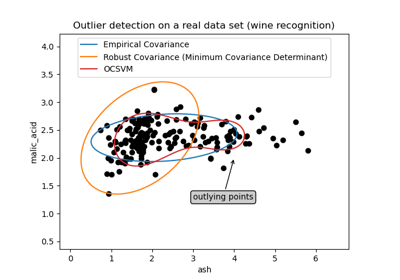Outlier detection on a real data set

Outlier detection on a real data set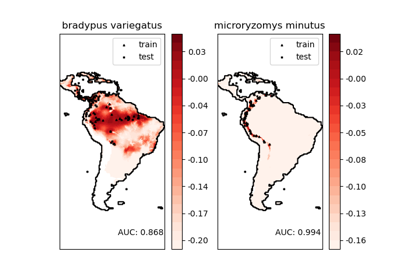Species distribution modeling

Species distribution modeling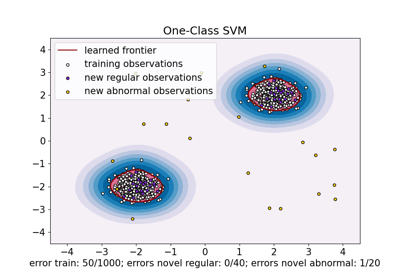One-Class SVM versus One-Class SVM using Stochastic Gradient Descent

One-Class SVM versus One-Class SVM using Stochastic Gradient Descent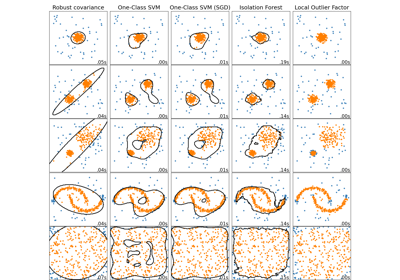Comparing anomaly detection algorithms for outlier detection on toy datasets

Comparing anomaly detection algorithms for outlier detection on toy datasets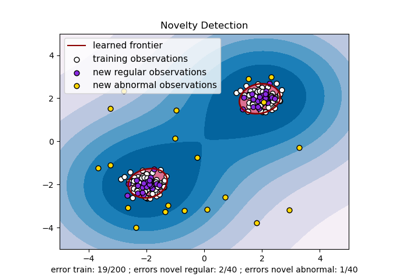One-class SVM with non-linear kernel (RBF)

One-class SVM with non-linear kernel (RBF)